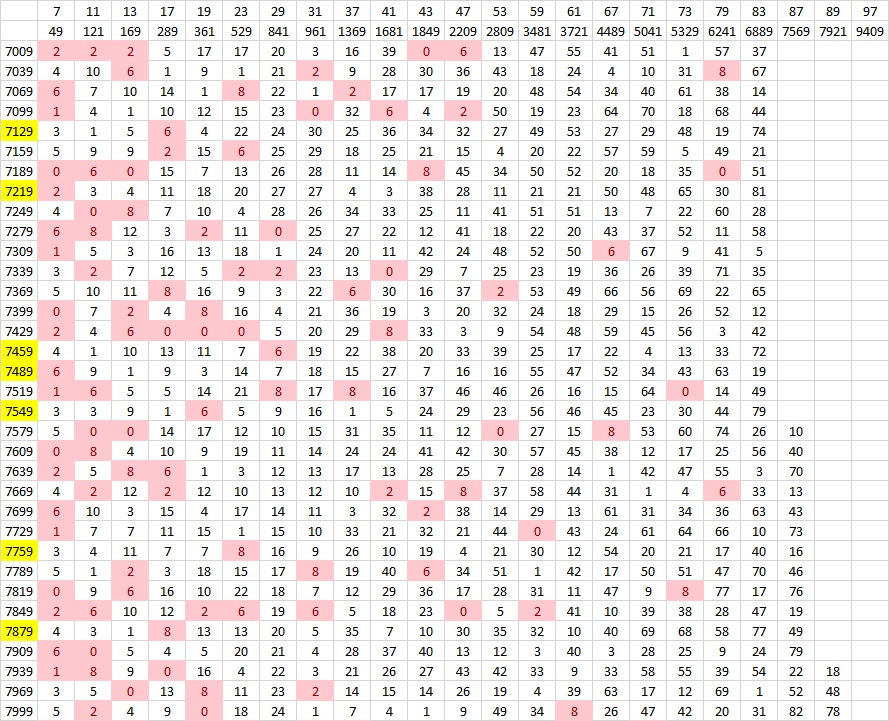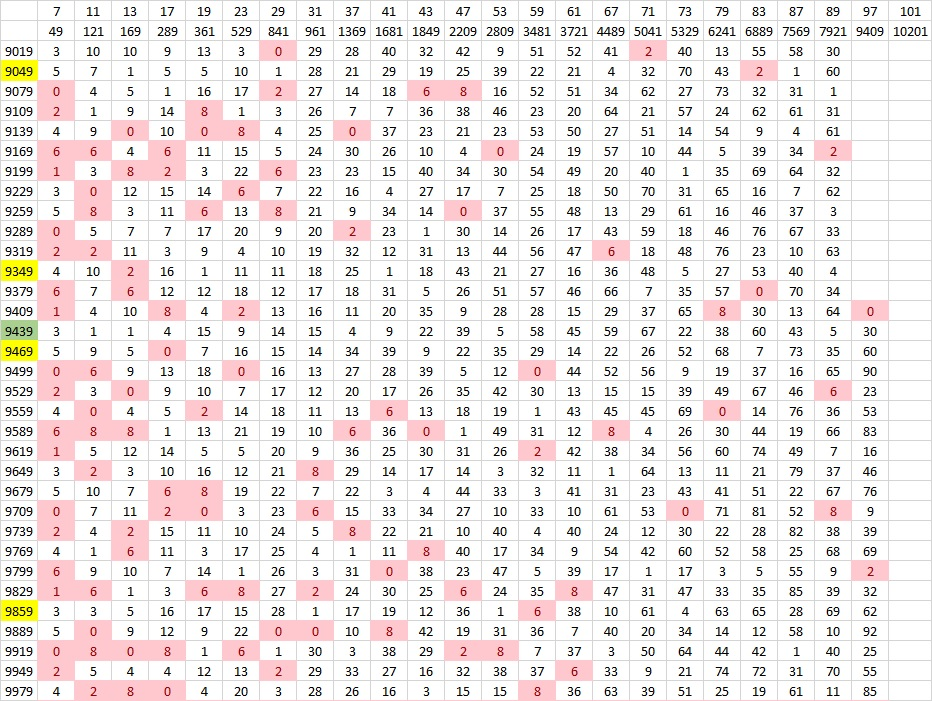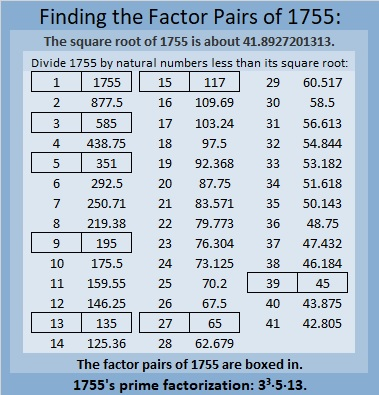# 1755 Searching for Prime Decades

Contents

### Today’s Puzzle:

Charles Sanders got me thinking about prime decades and how to find them. What a great puzzle for me to work on!

A prime decade is a set of four prime numbers that look the same except for their last digit.

The first decade has 4 prime numbers, 2, 3, 5, 7, but it is NOT a prime decade because 1 and 9 are not prime numbers. 5, 7, 11, 13 is the only prime quadruplet that is not also a prime decade. The first prime decade is 11, 13, 17, 19.

Could 1751, 1753, 1757, and 1759 form a prime decade? Since 1755 is divisible by 15, none of those other four odd numbers can be divisible by 3 or 5, so it is worth looking into if they are all prime numbers. We don’t have to test all four numbers, however, we only need to test 1759 using modular arithmetic. If we note the remainder when we divide 1759 by each prime number from 7 to 41, we will know. (We only have to go to 41 because √1759 < 43, so we don’t need any prime number divisor greater than or equal to 43.)

Will any remainder be a red flag? Yes, if any remainder is 0, 2, 6, or 8, then one of those four odd numbers won’t be prime. Also, if we divide 1759 by 7 and get a remainder of 1, then 1758 and 1751 will both be divisible by 7, and we won’t have a prime decade.

I did the divisions and everything looked great except
1759 ÷ 7 has a remainder of 2, so 1759 – 2 or 1757 is divisible by 7, and
1759 ÷ 17 has a remainder of 8, so 1759 – 8 or 1751 is divisible by 17.

In general, to find prime decades we only need to test the last number of the decades and we only need to test decades if the last number minus 4 is divisible by 15. I made a chart showing only those last numbers, and they appear in the first column. The top two rows are the prime number divisors and their squares. We will divide the last number in the decades by the prime numbers whose square is less than that last number. The remainders in pink are red flags. That would be a 1 in the 7’s column, and 0, 2, 6, or 8 in any column. Any of those would disqualify a decade from being a prime decade. 🙁

The green last numbers (in the first column) show the last number of actual prime decades. 🙂
The yellow last numbers have only ONE divisor that disqualifies the decade. 😐

What are the prime decades in this first millennia?As you can see, decades ending in 19, 109, 199, and 829 are all prime decades. This chart can also be useful in finding prime triplets because at least two of the prime numbers in a prime triplet whose first prime is greater than 10 must come from a decade whose middle number is divisible by 15. If a decade has two prime triplets in it that meet that qualification, it is also a prime decade.

In the next graphic, we see that the decades ending in 1489 and 1879 are prime decades. Here we also test the decade in which our post number, 1755, appears. We see 1759 has remainders of 2 and 8, confirming that 1757 and 1751 are not prime numbers.There is only one prime decade, 2081, 2083, 2087, 2089, in the next set of decades, and only one other decade that even came close to being a second one.There are two prime decades in the next group and several close calls:None in the next group, but there are several decades that ALMOST qualified.There’s a prime decade is in this group as well as a handful of decades that almost qualified.In this next group, there are only two decades that even came close to qualifying.The next two millennia have no prime decades but they do have several decades that came close to qualifying.Finally, we get another prime decade in this next group! (We would have had one right away if only 9047 wasn’t divisible by 83.)I could go on literally forever, but I’ll stop now. Instead, I’ll write a little more about the post number, 1755.

### Factors of 1755:

• 1755 is a composite number.
• Prime factorization: 1755 = 3 × 3 × 3 × 5 × 13, which can be written 1755 = 3³ × 5 × 13.
• 1755 has at least one exponent greater than 1 in its prime factorization so √1755 can be simplified. Taking the factor pair from the factor pair table below with the largest square number factor, we get √1755 = (√9)(√195) = 3√195.
• The exponents in the prime factorization are 3,1 and 1. Adding one to each exponent and multiplying we get (3 + 1)(1 + 1) (1 + 1) = 4 × 2 × 2 = 16. Therefore 1755 has exactly 16 factors.
• The factors of 1755 are outlined with their factor pair partners in the graphic below.### More About the Number 1755:

1755 is the hypotenuse of FOUR Pythagorean triples:
432-1701-1755, which is 27 times (16-63-65)
675-1620-1755, which is (5-12-13) times 135,
891-1512-1755, which is 27 times (33-56-65), and
1053-1404-1755, which is (3-4-5) times 351.

## 3 thoughts on “1755 Searching for Prime Decades”

1.Charles Sanders

Ma’am: thank you for your exposition. I started with the prime quadruplet {p, p+2, p+4, p+6}. To assure against a prime quintuplet, we require (p+4) congruent to 0 mod 5. If we add the integers in the index set together, we arrive at 2^2(p+4). In order that p+4 is divisible by 5, while remaining prime itself, then p must be of the form 30k+11, where k is the exact integer which makes p prime. Our index set becomes {30k+11, 30k+13, 30k+17, 30k+19}. As you have discovered for yourself, then comes a lot of down and dirty calculations. These are definitely speeded up by modular arithmetic. If you take the number p+4, the 4 remaining integers yield {-4, -2, 2, 4} mod (p+4). The residues sum to 0. Then each prime below the square root of (p+8) must follow the template already engendered by mod (p+4). All of these separate residues will also sum to 0, meaning there are no multiples of any primes in the decade of the prime quadruplet, save for the composite number p+4. This proves one has a prime decade QED. It gives one the precise tools to verify where an integer quadruplet is prime or not. The downside is that it cannot predict when the next quadruplet will occur. That was the formula I was seeking. It doesn’t exist.

•ivasallay

I don’t think we can escape those “down and dirty calculations.”

2.Charles Sanders

I said a prime less than the square root of p+8. I meant the first prime after that square root.

This site uses Akismet to reduce spam. Learn how your comment data is processed.